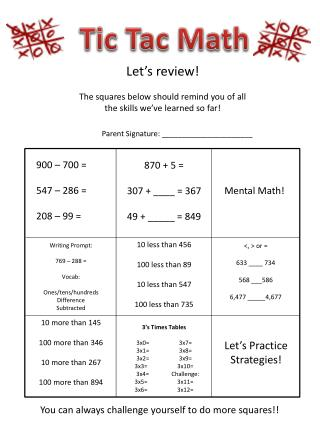DownloadDownload PresentationTic Tac Math

# Tic Tac Math

Download Presentation## Tic Tac Math

- - - - - - - - - - - - - - - - - - - - - - - - - - - E N D - - - - - - - - - - - - - - - - - - - - - - - - - - -
##### Presentation Transcript

1. Tic Tac Math Let’s review! The squares below should remind you of all the skills we’ve learned so far! Parent Signature: ______________________ 900 – 700 = 547 – 286 = 208 – 99 = 870 + 5 = 307 + ____ = 367 49 + _____ = 849 Mental Math! 10 less than 456 100 less than 89 10 less than 547 100 less than 735 Writing Prompt: 769 – 288 = Vocab: Ones/tens/hundreds Difference Subtracted <, > or = 633 ____ 734 568 ___586 6,477 _____4,677 10 more than 145 100 more than 346 10 more than 267 100 more than 894 3’s Times Tables 3x0= 3x7= 3x1= 3x8= 3x2= 3x9= 3x3= 3x10= 3x4= Challenge: 3x5= 3x11= 3x6= 3x12= Let’s Practice Strategies! You can always challenge yourself to do more squares!!